Skip to content
Related Articles
ML | K-Medoids clustering with solved example
• Difficulty Level : Easy
• Last Updated : 07 Aug, 2020

K-Medoids (also called as Partitioning Around Medoid) algorithm was proposed in 1987 by Kaufman and Rousseeuw. A medoid can be defined as the point in the cluster, whose dissimilarities with all the other points in the cluster is minimum.

The dissimilarity of the medoid(Ci) and object(Pi) is calculated by using E = |Pi - Ci|

The cost in K-Medoids algorithm is given asAlgorithm:

1. Initialize: select k random points out of the n data points as the medoids.
2. Associate each data point to the closest medoid by using any common distance metric methods.
3. While the cost decreases:
For each medoid m, for each data o point which is not a medoid:
1. Swap m and o, associate each data point to the closest medoid, recompute the cost.
2. If the total cost is more than that in the previous step, undo the swap.

Let’s consider the following example: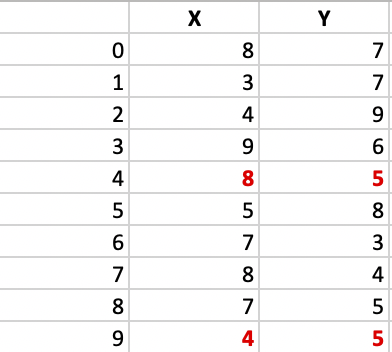If a graph is drawn using the above data points, we obtain the following: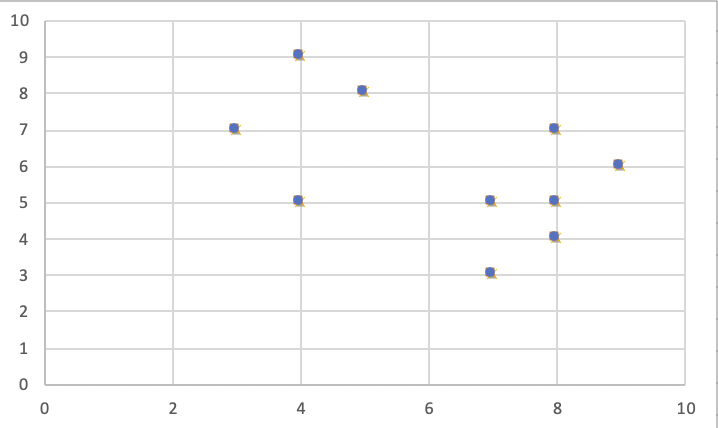Step 1:
Let the randomly selected 2 medoids, so select k = 2 and let C1 -(4, 5) and C2 -(8, 5) are the two medoids.

Step 2: Calculating cost.
The dissimilarity of each non-medoid point with the medoids is calculated and tabulated: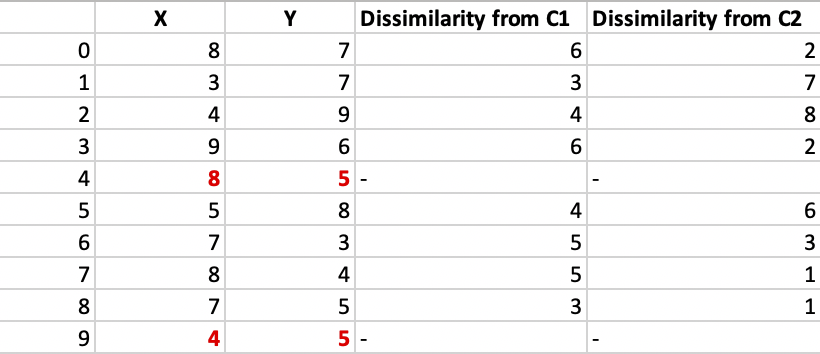Each point is assigned to the cluster of that medoid whose dissimilarity is less.
The points 1, 2, 5 go to cluster C1 and 0, 3, 6, 7, 8 go to cluster C2.
The Cost = (3 + 4 + 4) + (3 + 1 + 1 + 2 + 2) = 20

Step 3: randomly select one non-medoid point and recalculate the cost.
Let the randomly selected point be (8, 4). The dissimilarity of each non-medoid point with the medoids – C1 (4, 5) and C2 (8, 4) is calculated and tabulated.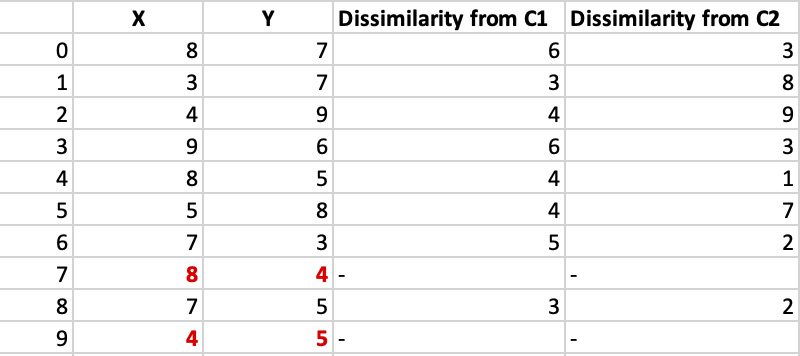Each point is assigned to that cluster whose dissimilarity is less. So, the points 1, 2, 5 go to cluster C1 and 0, 3, 6, 7, 8 go to cluster C2.
The New cost = (3 + 4 + 4) + (2 + 2 + 1 + 3 + 3) = 22
Swap Cost = New Cost – Previous Cost = 22 – 20 and 2 >0

As the swap cost is not less than zero, we undo the swap. Hence (3, 4) and (7, 4) are the final medoids. The clustering would be in the following way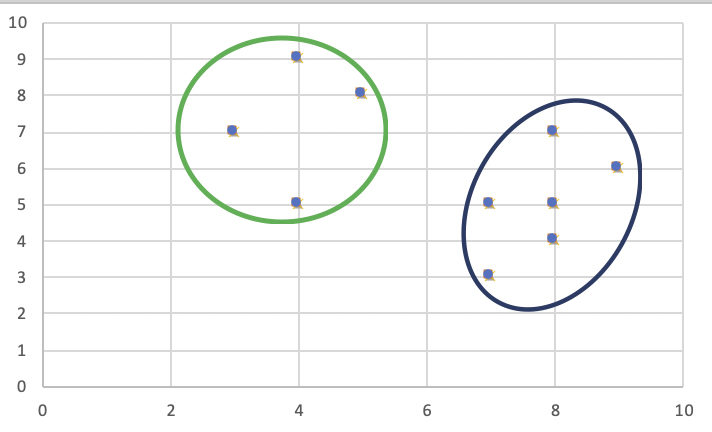The time complexity is.

Advantages:

1. It is simple to understand and easy to implement.
2. K-Medoid Algorithm is fast and converges in a fixed number of steps.
3. PAM is less sensitive to outliers than other partitioning algorithms.

Disadvantages:

1. The main disadvantage of K-Medoid algorithms is that it is not suitable for clustering non-spherical (arbitrary shaped) groups of objects. This is because it relies on minimizing the distances between the non-medoid objects and the medoid (the cluster centre) – briefly, it uses compactness as clustering criteria instead of connectivity.
2. It may obtain different results for different runs on the same dataset because the first k medoids are chosen randomly.My Personal Notes arrow_drop_up